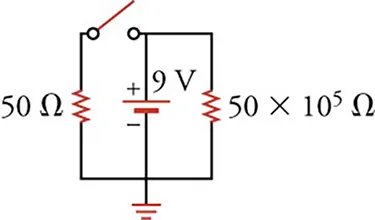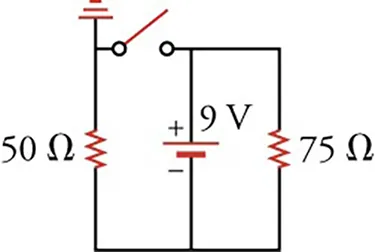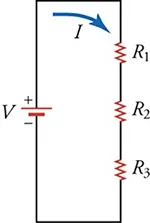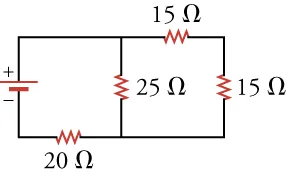Physics

#### 19.1Ohm's law

44.

True or false—it is possible to produce nonzero DC current by adding together AC currents.

1. false
2. true
45.

What type of current is used in cars?

1. alternating current
2. indirect current
3. direct current
4. straight current
46 .
If current were represented by $C$, voltage by $B$, and resistance by $g$, what would the mathematical expression be for Ohm’s law?
1. $C = Bg$
2. $g = BC$
3. $\frac{B}{C} = \frac{C}{g}$
4. $B = Cg$
47 .
Give a verbal expression for Ohm’s law.
1. Ohm’s law says that the current through a resistor equals the voltage across the resistor multiplied by the resistance of the resistor.
2. Ohm’s law says that the voltage across a resistor equals the current through the resistor multiplied by the resistance of the resistor.
3. Ohm’s law says that the resistance of the resistor equals the current through the resistor multiplied by the voltage across a resistor.
4. Ohm’s law says that the voltage across a resistor equals the square of the current through the resistor multiplied by the resistance of the resistor.
48.

What is the current through a 100-Ω resistor with 12 V across it?

1. 0
2. 0.12 A
3. 8.33 A
4. 1,200 A
49 .
What resistance is required to produce $0.15\,\text{A}$ from a $9.0\,\text{V}$ battery?
1. $0.017$
2. 1
3. 60
4. 120

#### 19.2Series Circuits

50.

Given a circuit with one 9-V battery and with its negative terminal connected to ground. The two paths are connected to ground from the positive terminal: the right path with a 20-Ω and a 100-Ω resistor and the left path with a 50-Ω resistor. How much current will flow in the right branch?

1. $9 120 9 120$
2. $9 100 9 100$
3. $9 50 9 50$
4. $9 20 9 20$
51 .

Through which branch in the circuit below does the most current flow?1. All of the current flows through the left branch due to the open switch.
2. All of the current flows through the right branch due to the open switch in the left branch.
3. All of the current flows through the middle branch due to the open switch in the left branch
4. There will be no current in any branch of the circuit due to the open switch.
52 .

What current flows through the $75\text{-}\Omega$ resistor in the circuit below?1. $0.072\,\text{A}$
2. $0.12\,\text{A}$
3. $0.18\,\text{A}$
4. $0.3\,\text{A}$
53 .
What is the resistance in the circuit below if the voltage source is a 9.0 V battery and the current is 0.25 A?1. 6.8 Ω
2. 12 Ω
3. 36 Ω
4. 72 Ω

#### 19.3Parallel Circuits

54.

Ten 100-Ω resistors are connected in series. How can you increase the total resistance of the circuit by about 40 percent?

1. Adding two 10-Ω resistors increases the total resistance of the circuit by about 40 percent.
2. Removing two 10-Ω resistors increases the total resistance of the circuit by about 40 percent.
3. Adding four 10-Ω resistors increases the total resistance of the circuit by about 40 percent.
4. Removing four 10-Ω resistors increases the total resistance of the circuit by about 40 percent.
55 .
Two identical resistors are connected in parallel across the terminals of a battery. If you increase the resistance of one of the resistors, what happens to the current through and the voltage across the other resistor?
1. The current and the voltage remain the same.
2. The current decreases and the voltage remains the same.
3. The current and the voltage increases.
4. The current increases and the voltage remains the same.
56.In the circuit shown, through which resistor(s) does the most current flow? Through which does the least flow? Explain.

1. The most current flows through the 15-Ω resistor because all the current must pass through this resistor.
2. The most current flows through the 20-Ω resistor because all the current must pass through this resistor.
3. The most current flows through the 25-Ω resistor because it is the highest resistance.
4. The same current flows through the all the resistor because all the current must pass through each of the resistors.

#### 19.4Electric Power

57.

You want to increase the power dissipated in a circuit. You have the choice between doubling the current or doubling the resistance, with the voltage remaining constant. Which one would you choose?

1. doubling the resistance
2. doubling the current
58.

You want to increase the power dissipated in a circuit. You have the choice between reducing the voltage or reducing the resistance, with the current remaining constant. Which one would you choose?

1. reduce the voltage to increase the power
2. reduce the resistance to increase the power
59.

What power is dissipated in the circuit consisting of 310-Ω resistors connected in series across a 9.0-V battery?

1. The power dissipated is 2430 W.
2. The power dissipated is 270 W.
3. The power dissipated is 2.7 W.
4. The power dissipated is 0.37 W.
60.

What power is dissipated in a circuit consisting of three 10-Ω resistors connected in parallel across a 9.0-V battery?

1. The power dissipated is 270 W.
2. The power dissipated is 30 W.
3. The power dissipated is 24 W.
4. The power dissipated is 1/24 W.
Order a print copy

As an Amazon Associate we earn from qualifying purchases.